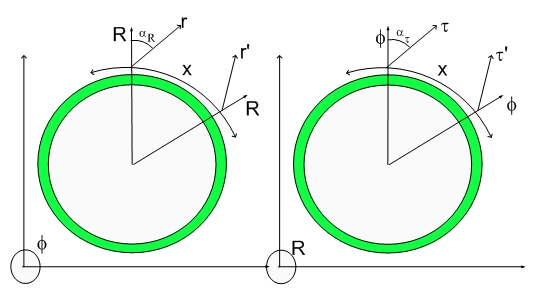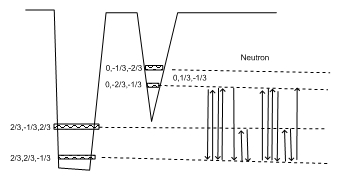# The Mass of a NeutrinoThe Mass of a Neutrino

Einstein's paradigm of a 4D Spacetime was later patched up with compact curled spaces - one for each new unnecessary quantum number (Strangeness, Bottom-Up etc) scientists introduced into their Standard Model...:)

The resulting patched Spacetime is still basically a 4D Spacetime in nature, that is, if there is a 3D point where the Universe started (Big Bang), then that same universe can return (Big Crunch) under the force of Gravitation...:)

That Big Crunch depends upon the sum of all masses and that includes those ubiquitous neutrinos...:)

Much speculation, calculations etc has been done on that subject.

Let me tell you my view of it...:)

As I mentioned in the Galileo Biggest Blunder, inertial mass and Gravitational Mass have been inadvertently made equal in the Galileo' Equivalence Principle.

In my theory that is not the case. Inertial and Gravitational Masses are only the same for standard matter...:) stuff composed of protons, electrons and neutrons.

Inertial mass is associated with the resistance to deformation of Fabric of Space on the footprints of matter (dilators).

Gravitational mass is modeled in my theory as the 4D displacement volume associated with dilator's cycles. This means that one can have a footprint on the 3D Fabric of Space and have ZERO GRAVITATIONAL MASS. This will always be the case if the frequency of the dilaton fields are not the same as the fundamental dilator frequency.

Every time someone says something about being in phase or having a frequency one has to mention what kind of clock is being used. Of course, proper time is not a good clock...;) The Tau vector (direction of Proper Time) depends upon local deformation of the Fabric of Space. This makes it clear that the only clock one can use is the Cosmological Time which is visible in both cross-sections of the Lightspeed Expanding Hyperspherical Universe shown above.

To understand what one means by frequency just analyse the Neutron Coherence Diagram below:One can easily recognize the Electron and Proton sub-coherences. The remaining steps correspond to rotations within the 3D space. Notice that the two states involved (2/3,-1/3,2/3) and (2/3,2/3,-1/3) differ just by a 90 rotation around the x axis. Four rotations will bring the deformational state back into its original state.

This is the Electron Neutrino - a coherence between two states of the Proton.

The Transmutation Sub-Coherences or Chords (red lines in this case) creates the needed delay between spinning and tunneling processes such as to move the electron state into a proton state and vice-versa. The resulting coherence has both negative and positive "charges", thus is a Neutron.

The decaying of a Neutron frees the red lines or Electron Neutrino. Since the rotation in a 3D space frequency doesn't match the tunneling frequency of the Fundamental Dilator Coherence, the neutrino interaction will average out to ZERO at any distance that is not really small...:)

That is the reason for the ethereal nature of Neutrinos...

Cheers,

MP

Currently unrated

### New Comment

required

required (not published)

optional

required